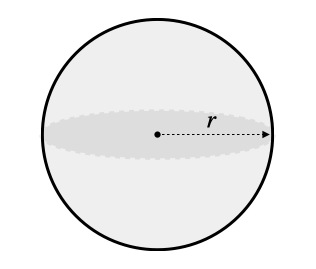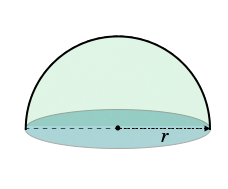## Introduction

• A sphere is a rounded three-dimensional solid.
• It is perfectly symmetrical.
• All the points on its surface are at an equal distance from its center. This distance is called the radius of the sphere.• Half of a sphere is called a hemisphere.## Volume of a Sphere

• If the radius $\left(r\right)$ of a sphere is known, its volume can be found by using the following formula:

• If the diameter $\left(d\right)$ of a sphere is known, its volume can be found by using the following formula:

• The volume of a sphere is measured in cubic units.

## Surface Area of a Sphere

• The surface area of a sphere is the total area covered by its outer surface.
• If the radius $\left(r\right)$ of a sphere is known, its surface area can be calculated using the formula mentioned below.

$Are{a}_{sphere}=4{\mathrm{\pi r}}^{2}$

## Solved Examples

Question 1: Find the volume of a sphere with a radius of 3 cm.

Solution:

Question 2: If the radius of a sphere is 20 cm, find its surface area.

Solution: $Are{a}_{sphere}=4{\mathrm{\pi r}}^{2}$

## Cheat Sheet

• $Are{a}_{sphere}=4{\mathrm{\pi r}}^{2}$

## Blunder Areas

• The curved surface area and total surface area of a sphere are the same. But the curved surface area and total surface of a hemisphere are not the same.